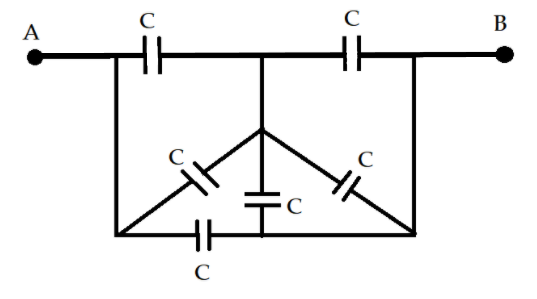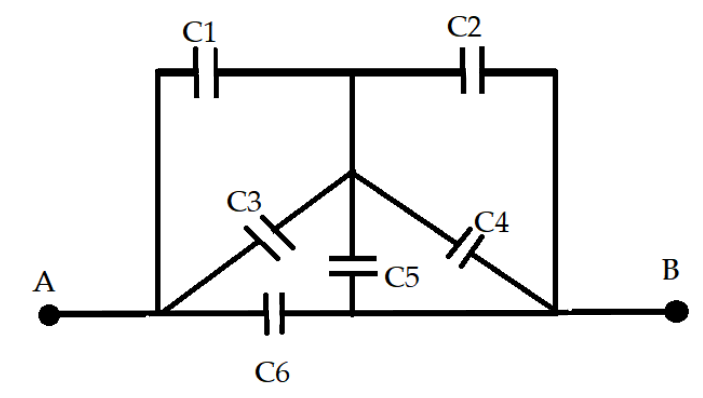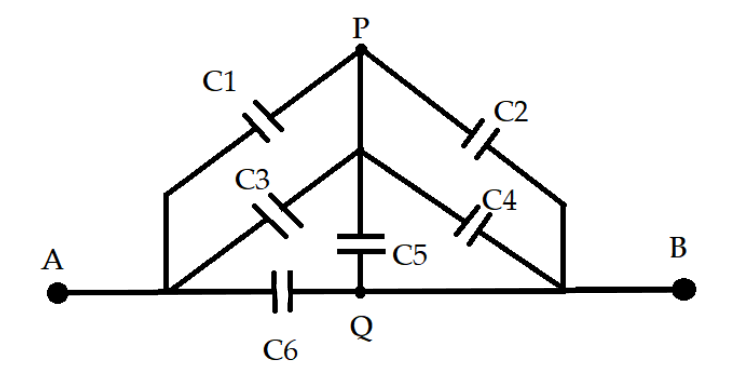QuestionAnswers

# In the given network of capacitors, the equivalent capacitance between point $A$ and $B$ isa. $\dfrac{6}{5}C$b. $2C$c. $\dfrac{9}{{11}}C$d. $\dfrac{{11}}{5}C$Verified
91.5k+ views
Hint: Here in this question capacitors are connected in different arrangements in a circuit between two given points. A capacitor is generally an electronic device that stores electrical energy in the electric field and it is a passive electronic component as it receives energy.

Formula used:
Parallel connection of the capacitors
${C_T} = {C_A} + {C_B} + ....$
Series connection of the capacitors
${C_T} = \dfrac{{{C_A} \times {C_B} \times ..}}{{{C_A} + {C_B} + ..}}$

We will start with the different arrangements of the components and analyze whether which are connected in series connection or parallel connection and then they are calculated separately and then the equivalence capacitor is then evaluated.Staring with the rearrangements of the points $A$ and $B$ given on the circuits. All the capacitors have the same capacitance
${C_1} = {C_2} = {C_3} = {C_4} = {C_5} = {C_6} = C$From the figure, it can be concluded that the capacitors ${C_1}$ and ${C_3}$ are in the parallel connection between points $P$ , and $A$ hence their equivalent capacitance is given as
${C_{AP}} = {C_1} + {C_3}$
Substitute the values of ${C_1}$and ${C_3}$ hence,
${C_{AP}} = C + C$
$\Rightarrow {C_{AP}} = 2C$
Similarly, the between points $P$ and $B$ the capacitors ${C_2}$,${C_4}$ and ${C_5}$ are in parallel connection hence their equivalent capacitance is given as
${C_{BP}} = {C_2} + {C_4} + {C_5}$
$\Rightarrow {C_{BP}} = C + C + C = 3C$

Now on analyzing, it is found that the capacitor with $3C$ and $2C$ are in series hence their equivalent capacitance is given as
$C''' = \dfrac{{3C \times 2C}}{{3C + 2C}}$
$\Rightarrow C''' = \dfrac{{6C}}{5}$
Hence the final equivalent capacitance between the points $A$ and $B$ can be given by the parallel connection between $C'''$ and $C$ which is given as
${C_{AB}} = \dfrac{{6C}}{5} + C$
$\Rightarrow {C_{AB}} = \dfrac{{6C + 5C}}{5} = \dfrac{{11}}{5}C$
Hence the final equivalent capacitance between the points $A$ and $B$ can be given as $\dfrac{{11}}{5}C$.

Hence, the correct answer is option (D).

Note: Here it is noted that the equivalence formula of capacitors is different from the other electronic components like resistance. Hence one should not get confused between the equivalence formula between resistance and capacitors. For resistance connected in series, the equivalence formula is given by $R = {R_1} + {R_2} + ...$ , and for resistance connected in parallel, the formula is given as $R = \dfrac{{{R_1}{R_2}...}}{{{R_1} + {R_2} + ..}}$.